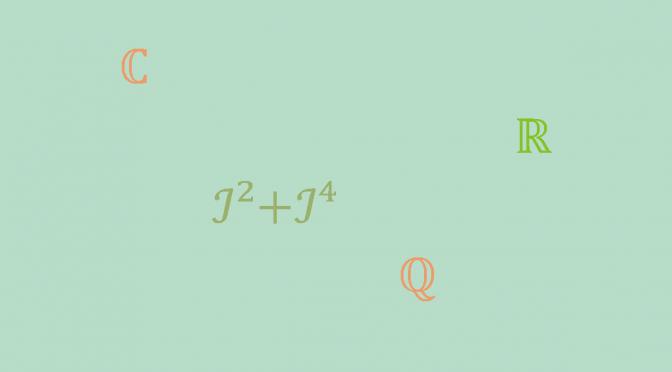An infinite field that cannot be ordered

Introduction to ordered fields

Let $$K$$ be a field. An ordering of $$K$$ is a subset $$P$$ of $$K$$ having the following properties:

ORD 1
Given $$x \in K$$, we have either $$x \in P$$, or $$x=0$$, or $$-x \in P$$, and these three possibilities are mutually exclusive. In other words, $$K$$ is the disjoint union of $$P$$, $$\{0\}$$, and $$-P$$.
ORD 2
If $$x, y \in P$$, then $$x+y$$ and $$xy \in P$$.

We shall also say that $$K$$ is ordered by $$P$$, and we call $$P$$ the set of positive elements.

Let’s notice following properties of an ordered field:

• Since $$1 \neq 0$$ and $$1=1^2=(-1)^2$$ we see that $$1 \in P$$.
• More generally, a non zero square is always positive because for $$x \neq 0$$, $$x^2=(-x)^2$$ and either $$x \in P$$ or $$-x \in P$$.

A finite field cannot be ordered

Let us assume that $$K$$ is ordered by $$P$$. By ORD 2, it follows that $$p= 1+ \cdots + 1 \in P$$, whence $$K$$ has characteristic $$0$$ and therefore cannot be finite.

$$\mathbb{Q}$$ is an infinite ordered field

One can verify at once that $$\mathbb{Q}$$ is ordered by taking for  $$P$$ the set of positive rational numbers.

This is the only possible ordering of $$\mathbb{Q}$$. Suppose that $$\mathbb{Q}$$ is ordered by $$T$$. We have seen above that $$1 \in T$$. Therefore $$n=1 + \cdots +1 \in P$$, i.e. $$\mathbb{N^*} \subset T$$.
If $$m$$ is a positive integer, $$\frac{1}{m}=m \cdot \frac{1}{m^2} \in T$$ by ORD 2 and consequently $$\mathbb{Q}^*_+ \subset T$$. A negative rational cannot belong to $$T$$ because $$0$$ would also belong to $$T$$ in contradiction with ORD 1. Finally $$T=\mathbb{Q}^*_+$$.

$$\mathbb{C}$$ is an infinite field that cannot be ordered

While $$\mathbb{Q}$$ is an ordered field included in $$\mathbb{C}$$, $$\mathbb{C}$$ cannot be ordered. To show that this is the case, assume that $$\mathbb{C}$$ is ordered by a subset $$P$$. If $$\imath \in P$$, then by ORD 2, $$\imath^2 = -1 \in P$$ and $$\imath^4 = 1 \in P$$. Still according to ORD 2, $$\imath^2 + \imath^4=0 \in P$$ contradicting ORD 1. And if $$-\imath \in P$$, we have the same contradiction as before because $$(-\imath)^2 = \imath^2$$.